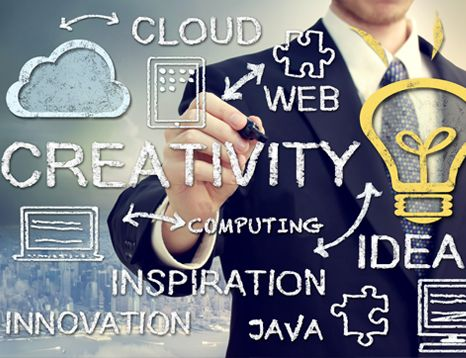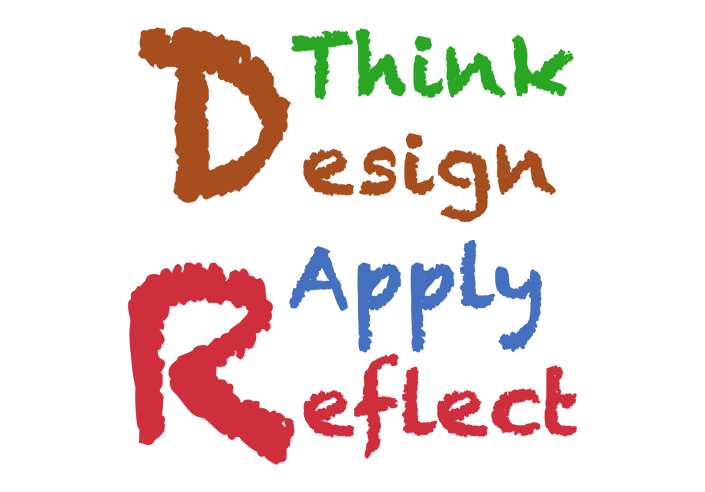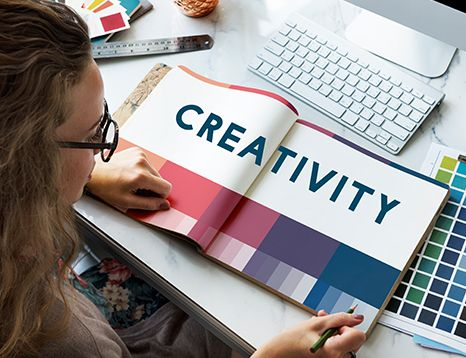## Introduction to Creative Computing - I

• Skill Level: Intermediate
• Prerequisite: Introduction to Computer Science
• Software: JavaScript and Java
• Duration: 3 Quarters. Each Quarter is 12 Weeks.

## Course DescriptionCreative Computing introduces students to the formal structure and syntax of the programming language.Here students learn syntax for the computational and logical concepts they have developed while working with blocks.This course is broken down in a stair case manner into 3 quarters, where each quarter builds on the work of the previous quarter. All the concepts and applications in this course are taught using the concrete-visual-abstract approach guided by psychologist Jerome Bruner’s theory of intellectual development.Course starts with students working on smaller projects at the start of the quarter and culminates each quarter with a complex and challenging project. In this course students use logic, problem solving and abstraction to create various computational artifacts like: logos, animations,games, applications and visualizations. This is a cross-curricular course where in addition to computer science, students are also taught fundamental concepts of mathematics and physics.Students learn how information around them is digitized, stored and used. By the end of this course students will be able to create simple application, animations and games using JavaScript and Java. Additionally, students will be able to simulate basic principles of motion physics.

### 12 Weeks

During this quarter students are introduced to the formal structure of the programming language. Students explore and work with drawing primitives, data, variables, functions, frames, scenes, coordinate planes, arcs, angles, scaling, proportions and relative positions.

### 12 Weeks

During this quarter students work with various logical structures: conditionals, loops and functions. Students also start interacting with various IO elements and write applications to show there understanding.

### 12 Weeks

Durng this quarter students start working with arrays, rotations, circular motion, basic trigonometry, motion physics, and algorithms.This quarter students work on projects that span multiple sessions.

## What will students learn?

This course introduced students to algorithms, logic, conditionals and abstract thinking by creating simple animations and games.

## Goal of this course is to:

• Foster creativity
• Entool students to become makers of technology
• Build Confidence
• Help make Computer Science a tool of expression for young children.
• Learn what is computer science.## Skill Students Gain

• Computational Thinking
• Sequencing
• Problem solving
• Algorithming Thinking
• Understanding and Application of Logical Structures: conditionals and repetitions